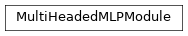# `garage.torch.modules.multi_headed_mlp_module`¶

class MultiHeadedMLPModule(n_heads, input_dim, output_dims, hidden_sizes, hidden_nonlinearity=torch.relu, hidden_w_init=nn.init.xavier_normal_, hidden_b_init=nn.init.zeros_, output_nonlinearities=None, output_w_inits=nn.init.xavier_normal_, output_b_inits=nn.init.zeros_, layer_normalization=False)

Bases: `torch.nn.Module`A PyTorch module composed only of a multi-layer perceptron (MLP) with multiple parallel output layers which maps real-valued inputs to real-valued outputs. The length of outputs is n_heads and shape of each output element is depend on each output dimension

Parameters
• n_heads (int) – Number of different output layers

• input_dim (int) – Dimension of the network input.

• output_dims (int or list or tuple) – Dimension of the network output.

• hidden_sizes (list[int]) – Output dimension of dense layer(s). For example, (32, 32) means this MLP consists of two hidden layers, each with 32 hidden units.

• hidden_nonlinearity (callable or torch.nn.Module or list or tuple) – Activation function for intermediate dense layer(s). It should return a torch.Tensor. Set it to None to maintain a linear activation.

• hidden_w_init (callable) – Initializer function for the weight of intermediate dense layer(s). The function should return a torch.Tensor.

• hidden_b_init (callable) – Initializer function for the bias of intermediate dense layer(s). The function should return a torch.Tensor.

• output_nonlinearities (callable or torch.nn.Module or list or tuple) – Activation function for output dense layer. It should return a torch.Tensor. Set it to None to maintain a linear activation. Size of the parameter should be 1 or equal to n_head

• output_w_inits (callable or list or tuple) – Initializer function for the weight of output dense layer(s). The function should return a torch.Tensor. Size of the parameter should be 1 or equal to n_head

• output_b_inits (callable or list or tuple) – Initializer function for the bias of output dense layer(s). The function should return a torch.Tensor. Size of the parameter should be 1 or equal to n_head

• layer_normalization (bool) – Bool for using layer normalization or not.

forward(input_val)

Forward method.

Parameters

input_val (torch.Tensor) – Input values with (N, *, input_dim) shape.

Returns

Output values

Return type

List[torch.Tensor]